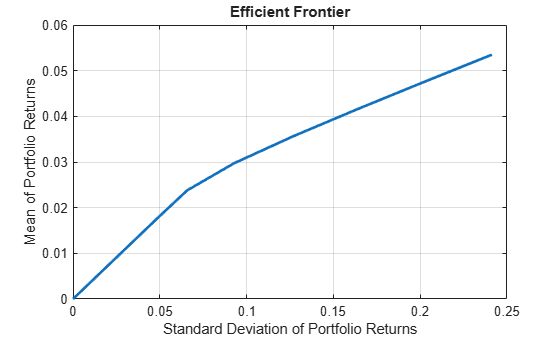abs2active

Convert constraints from absolute to active format

Description

example

ActiveConSet = abs2active(AbsConSet,Index) transforms a constraint matrix to an equivalent matrix expressed in active weight format (relative to the index).

Examples

collapse all

Set up constraints for a portfolio optimization for portfolio w0 with constraints in the form A*w <= b, where w is absolute portfolio weights. (Absolute weights do not depend on the tracking portfolio.) Use abs2active to convert constraints in terms of absolute weights into constraints in terms of active portfolio weights, defined relative to the tracking portfolio w0. Assume three assets with the following mean and covariance of asset returns:

m = [ 0.14; 0.10; 0.05 ];
C = [ 0.29^2 0.4*0.29*0.17 0.1*0.29*0.08; 0.4*0.29*0.17 0.17^2 0.3*0.17*0.08;...
0.1*0.29*0.08 0.3*0.17*0.08 0.08^2 ];

Absolute portfolio constraints are the typical ones (weights sum to 1 and fall from 0 through 1), create the A and b matrices using portcons.

AbsCons = portcons('PortValue',1,3,'AssetLims', [0; 0; 0], [1; 1; 1;]);

Use the Portfolio object to determine the efficient frontier.

p = Portfolio('AssetMean', m, 'AssetCovar', C);
p = p.setInequality(AbsCons(:,1:end-1), AbsCons(:,end));
p.plotFrontier;The tracking portfolio w0 is:

w0 = [ 0.1; 0.55; 0.35 ];

Use abs2active to compute the constraints for active portfolio weights.

ActCons = abs2active(AbsCons, w0)
ActCons = 8×4

1.0000    1.0000    1.0000         0
-1.0000   -1.0000   -1.0000         0
1.0000         0         0    0.9000
0    1.0000         0    0.4500
0         0    1.0000    0.6500
-1.0000         0         0    0.1000
0   -1.0000         0    0.5500
0         0   -1.0000    0.3500

Use the Portfolio object p and its efficient frontier to demonstrate expected returns and risk relative to the tracking portfolio w0.

p = p.setInequality(ActCons(:,1:end-1), ActCons(:,end));
p.plotFrontier;Note, when using abs2active to compute “active constraints” for use with a Portfolio object, don't use the Portfolio object’s default constraints because the relative weights can be positive or negative (the setDefaultConstraints function for a Portfolio object specifies weights to be nonnegative).

Input Arguments

collapse all

Portfolio linear inequality constraint matrix expressed in absolute weight format, specified as [A b] such that A*w <= b, where A is a number of constraints (NCONSTRAINTS) by number of assets (NASSETS) weight coefficient matrix, and b and w are column vectors of length NASSETS. The value w represents a vector of absolute asset weights whose elements sum to the total portfolio value. See the output ConSet from portcons for additional details about constraint matrices.

Data Types: double

Index portfolio weights, specified as a NASSETS-by-1 vector. The sum of the index weights must equal the total portfolio value (for example, a standard portfolio optimization imposes a sum-to-1 budget constraint).

Data Types: double

Output Arguments

collapse all

Transformed portfolio linear inequality constraint matrix expressed in active weight format, returned in the form [A b] such that A*w <= b. The value w represents a vector of active asset weights (relative to the index portfolio) whose elements sum to zero.

Algorithms

abs2active transforms a constraint matrix to an equivalent matrix expressed in active weight format (relative to the index). The transformation equation is

$A{w}_{absolute}=A\left({w}_{active}+{w}_{index}\right)\le {b}_{absolute}.$

Therefore

$A{w}_{active}\le {b}_{absolute}-A{w}_{index}={b}_{active}.$

The initial constraint matrix consists of NCONSTRAINTS portfolio linear inequality constraints expressed in absolute weight format. The index portfolio vector contains NASSETS assets.